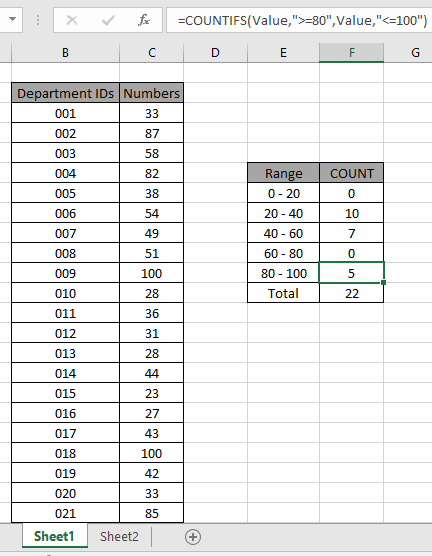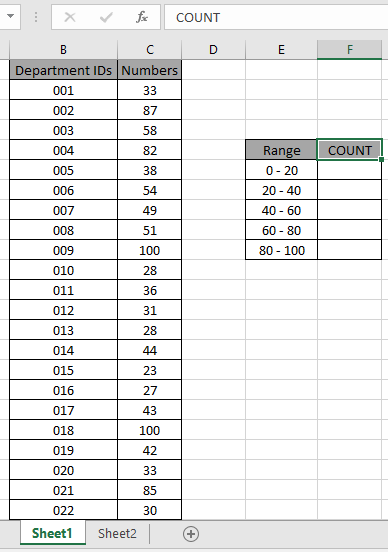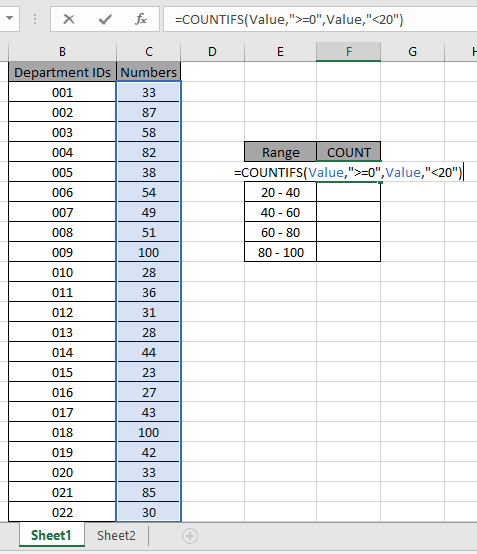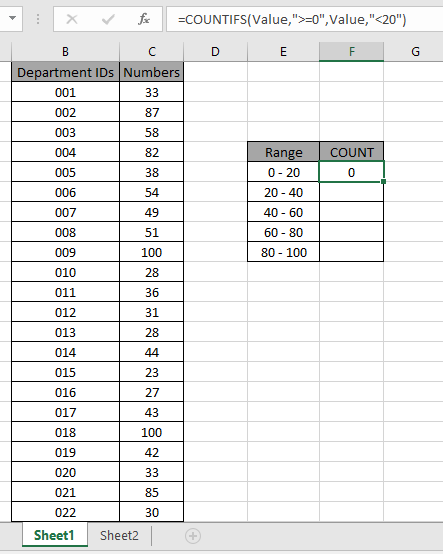# How to Count Between Given Range in ExcelMany times we get the need to count values between given ranges in Excel. For example, counting employees between 20-30 age, or employees who  have at least 3 and max 6 years of experience. All these are range criteria. In this article we will learn how to count values between given ranges using COUNTIFS function.

Generic formula:-

= COUNTIFS ( list , ">=low", list , "<high" )

List: list of numbers

Low: lower limit of the given range (included)

High: higher limit of the given range (excluded)

Example:

All of these might be confusing to understand. So, let's test this formula via running it on the example shown below.

Here we have the ID records and we need to count IDs where respective numbers lays between the given range.Number array given as Value name range for the array C3:C24.

Use the formula:

= COUNTIFS ( Value , ">=0" , Value , "<20" )

Explanation:

• The first criteria includes every number that is equal to or greater than 0.
• The second criteria excludes every number that is greater than or equal to 20. This leaves us with  numbers between 0 and 20.The formula looks like as shown in the above snapshot. Value array is given as named range.As you can see, the total values that occur between 0 - 20 comes out to be 0 i.e. there is no such value between 0 - 20.

Now copy the formula in other cells using the drag down option or using the shortcut key Ctrl + D as shown below. And changing As you can see the values that lay between 20 - 40 are 10.

Now calculate all the other counts with the given range values as explained above.We obtained all the count between given range as it also proves the formula works fine.

Here are some observational notes shown below.

Notes:

1. The formula only works with both numbers and text both.
2. The formula ignores text values while comparing numbers and ignores numbers when matching text values.
3. Operators like equals to ( = ), less than equal to ( <= ), greater than ( > ) or not equals to ( <> ) can be performed within function applied with number only.

Hope this article about how to count cells by range in Excel is explanatory. Find more articles on COUNTIFS functions here.

If you liked our blogs, share it with your friends on Facebook. And also you can follow us on Twitter and Facebook. We would love to hear from you, do let us know how we can improve, complement or innovate our work and make it better for you. Write to us at info@exceltip.com

Related Articles

How to use the SUMPRODUCT function in Excel: Returns the SUM after multiplication of values in multiple arrays in excel.

COUNTIFS with Dynamic Criteria Range : Count cells dependent on other cell values in Excel.

COUNTIFS Two Criteria Matches: Count cells matching two different criteria on the list in excel.

COUNTIFS With OR For Multiple Criteria : Count cells that have multiple criteria that match using the OR function.

COUNTIFS Function in Excel : Count cells dependent on other cell values.

How to Use Countif in VBA in Microsoft Excel : Count cells using Visual Basic for Applications code.

How to use wildcards in excel : Count cells matching phrases using the wildcards in excel

Popular Articles

50 Excel Shortcuts to Increase Your Productivity

Edit a dropdown list

Absolute reference in Excel

If with conditional formatting

If with wildcards

Vlookup by date

Terms and Conditions of use

The applications/code on this site are distributed as is and without warranties or liability. In no event shall the owner of the copyrights, or the authors of the applications/code be liable for any loss of profit, any problems or any damage resulting from the use or evaluation of the applications/code.